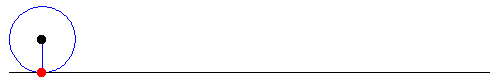# Newtonian Mechanics 04

— 1. Variational Calculus —

 Definition 1 A functional is a mapping from vector spaces to into real numbers.

Let$J = \int _{x_1}^{x^2} f\{y(x),y\prime (x),x\}dx$. Suppose that${x_1}$ and${x_2}$ are constants, the functional form of${f}$ is known.

According to definition 1${J}$ is a functional and the goal of the Calculus of Variations is to determine${y(x)}$ such that the value of${J}$ is an extremum.

Let${y=y(\alpha, x)}$ be a parametric representation of${y}$ such that${y(0,x)=y(x)}$ is the function that makes${J}$ an extremum.

We can write${y(\alpha, x)=y(0,x)+ \alpha\eta(x}$, where${\eta (x)}$ is a function of${x}$ of the class${C^1}$ (that means that${\eta}$ is a continuous function whose derivative is also continuous) with${\eta (x_1)=\eta (x_2)=0}$.

Now${J}$ is of the form$J(\alpha) = \int _{x_1}^{x^2} f\{y(\alpha, x),y\prime (\alpha, x),x\}dx$

Therefore the condition for${J}$ to be an extremum is$\displaystyle \frac{dJ}{d\alpha}(\alpha=0)=0$

 Example 1 Let${y(x)=x}$. Take${y(\alpha, x)= x+ \alpha\sin x}$ as a parametric representation of${y}$. Let${f=\left(dy/dx\right)^2}$,${x_1=0}$ and${x_2=2\pi}$. Given the previous parametric equation find${\alpha}$ such that${J}$ is a minimum. Now${\eta (0)=\eta (2\pi)=0}$ and${dy/dx=1+\alpha\cos x}$. Hence$J(\alpha)= \int_0^{2\pi}(1+2\alpha\cos x +\alpha^2\cos ^2x)dx=2\pi+\alpha^2\pi$. By the previous expression${J(\alpha)}$ it is trivial to see that the minimum value is reached when${\alpha=0}$
 Exercise 1 Given the points${(x_1,y_1)=(0,0)}$ and${(x_2,y_2)=(1,0)}$, calculate the equation of the curve that minimizes the distance between the points. Now${y(\alpha, x)=y(0,x)+\alpha \eta (x) = 0+\alpha(x^2-x)}$. It is${\eta (x) = x^2-x}$,${ds=\displaystyle \sqrt{dx^2+dy^2}=\sqrt{1+(dy/dx)^2}dx}$ And it is${s= \displaystyle \int _0^1 \sqrt{1+(dy/dx)^2}dx}$ with${dy/dx=\alpha (2x-1)}$. The rest is left as an exercise for the reader.

— 2. Euler Equations —

In the following section we’ll analyze the condition for${J}$ to be an extremum:{\begin{aligned} \frac{\partial J}{\partial \alpha} &= \frac{\partial}{\partial \alpha} \int _{x_1}^{x_2}f(y,y\prime,x)dx \\ &= \int _{x_1}^{x_2}\left(\frac{\partial f}{\partial y}\frac{\partial y}{\partial \alpha}+ \frac{\partial f}{\partial y\prime}\frac{\partial y\prime}{\partial \alpha}\right) dx \end{aligned}}

Since it is${\partial y /\partial \alpha = \eta (x)}$ and${\partial y\prime /\partial \alpha = d\eta/dx}$ it follows$\displaystyle \frac{\partial J}{\partial \alpha}= \int _{x_1}^{x_2}\left(\frac{\partial f}{\partial y}\eta (x)+ \frac{\partial f}{\partial y\prime}\frac{d \eta}{dx}\right) dx$

Now$\int _{x_1}^{x_2}\frac{\partial f}{\partial y\prime}\frac{d \eta}{dx}dx=\frac{\partial f}{\partial y\prime}\eta (x)|_{x_1}^{x_2}- \int _{x_1}^{x_2}\frac{d}{dx}\left( \frac{\partial f}{\partial y\prime} \right)\eta (x) dx$.

For the first term it is${\frac{\partial f}{\partial y\prime}\eta (x)|_{x_1}^{x_2}=0}$ since${\eta (x_1)=\eta (x_2)=0}$ by hypothesis.

Hence{\begin{aligned} \frac{\partial J}{\partial \alpha} &= \int _{x_1}^{x_2}\left(\frac{\partial f}{\partial y}\frac{\partial y}{\partial \alpha}- \frac{d}{dx}\left( \frac{\partial f}{\partial y\prime} \right) \frac{\partial y}{\partial \alpha}\right)dx \\ &= \int _{x_1}^{x_2}\left( \frac{\partial f}{\partial y}-\frac{d}{dx}\frac{\partial f}{\partial y\prime} \right)\eta (x) dx \end{aligned}}

Remembering that${\partial J / \partial\alpha(\alpha=0)=0}$ and taking into account the fact that${\eta (x)}$ is an arbitrary function one can conclude that$\displaystyle \frac{\partial f}{\partial y}-\frac{d}{dx}\frac{\partial f}{\partial y\prime}=0$

The previous equation is known as the Euler’s Equation

 Example 2 As an example let us try to derive the equations of motion of a particle that moves in a constant force field starting its movement from the state of rest. The particles starts from point${x_1, y_1}$ and goes to point${x_2, y_2}$.From the enunciate it follows${K+U=c}$. Let us take our original point as being our reference point for the potential. Then it is${k+U=0}$. As always it is${k=1/2mv^2}$. For the potential it is${U=-Fx=-mgx}$. From the previous equations it follows that${v=\sqrt{2gx}}$. From the definition of velocity it follows that$\displaystyle t=\int _{x_1,y_1}^{x_2,y_2} \frac{ds}{v}=\int _{x_1,y_1}^{x_2,y_2}\frac{\sqrt{dx^2+dy^2}}{\sqrt{2gx}}=\int _{x_1,y_1}^{x_2,y_2}\frac{\sqrt{1+y\prime^2}}{\sqrt{2gx}}dx$ Let${f=\sqrt{\frac{1+y\prime^2}{x}}}$ since${(2g)^{-1/2}}$ is only a constant factor and can be omitted from our analysis. Given the functional form of${f}$ it is${df/dy=0}$ and Euler’s Equation just is:$\displaystyle \frac{d}{dx}\frac{\partial f}{\partial y\prime}=0$ From the previous relationship it is$\displaystyle \frac{\partial f}{\partial y\prime}=(2a)^{-1/2}=\mathrm{const}$ Hence it is{\begin{aligned} \frac{y\prime^2}{x(1+y\prime^2)} &= \frac{1}{2a} \Rightarrow\\ y &= \int \frac{x}{\sqrt{2ax-x^2}}dx \end{aligned}} Making the change of variables${x=a(1-\cos \theta)}$ it follows${dx=a\sin \theta d\theta}$. Hence the expression for${y}$ is${y=\int a(1-\cos \theta)d\theta\Rightarrow y=a(\theta-\sin \theta)+A}$. Since our particle starts from the origin it is${A=0}$. Thus the solution to our initial problem is{\begin{aligned} x &= a(1-\cos \theta) \\ y &= a(\theta-\sin \theta) \end{aligned}} Which are the parametric equations of a cycloid.Cycloid

To close our thoughts on the Euler equation let us say that there also is a second form for the Euler equation. The second form is$\displaystyle f-y\prime\frac{\partial f}{\partial y\prime}= \mathrm{const}$

and is used in the cases where${f}$ doesn’t depend explicitly on${x}$.

— 3. Euler Equation for${n}$ variables —

Let${f}$ be of the form${f=f\{ y_1(x),y\prime _1(x),y_2(x),y\prime _2(x),\cdots,y_n(x),y\prime _n(x), x \}}$.

Now we have${y_i(\alpha, x)= y_i(0,x)+\alpha \eta (x)}$ and$\int _{x_1}^{x_2}\left( \frac{\partial f}{\partial y_i}-\frac{d}{dx}\frac{\partial f}{\partial y _i\prime} \right)\eta _i (x) dx$ for each of the values of${i}$. Since${\eta _i(x)}$ are independent functions it follows that for${\alpha=0}$$\displaystyle \frac{\partial f}{\partial y_i}-\frac{d}{dx}\frac{\partial f}{\partial y _i\prime}=0$

That is to say we have${n}$ independent Euler equations.

## 10 comments on “Newtonian Mechanics 04”

1. […] function can be identified with the function that we saw on Newtonian Mechanics 04 if one makes the obvious […]

2. joeschmo26 says:

“Hence {\displaystyle J(\alpha)= \int_0^{2\pi}(1+\alpha\cos x +\alpha^2\cos ^2x)dx=2\pi+\alpha^2\pi}.”

Should the second term be${\2\alpha\cos x}$ ?

• joeschmo26 says:

“Hence$J(\alpha)= \int_0^{2\pi}(1+\alpha\cos x +\alpha^2\cos ^2x)dx=2\pi+\alpha^2\pi}$.”

Should the second term be${ 2\alpha\cos x }$ ?

• ateixeira says:

Yes it should. Sorry for the typo and thanks for the help.

3. joeschmo26 says:

In Example 2 where you derive the equations of motion for the cycloid… is${frac{\partial{f}}{\partial{y}} = 0}$ because${f}$ is not dependent on${y}$, or is there some other reason?

• joeschmo26 says:

Edit:${ frac{\partial f}{\partial y} = 0}$

• joeschmo26 says:

Attempt 3:${ \frac{\partial f}{\partial y} = 0}$

• ateixeira says:

That’s exactly the reason.

4. joeschmo26 says:

And I assume Exercise 1 ( without actually completing the entire integral) that the solution comes from trigonometric substitution which its second to last step is${\int \sec\theta d\theta} = ln( \sec\theta + \tan\theta )$ After evaluation with the limits in the theta domain the right side becomes 0, hence y = 0 is the equation of the line?

• ateixeira says:

You have to make a trigonometric change of variable to calculate this integral and then you need to make an approximation but it is slightly more complicated than what you present.

This site uses Akismet to reduce spam. Learn how your comment data is processed.